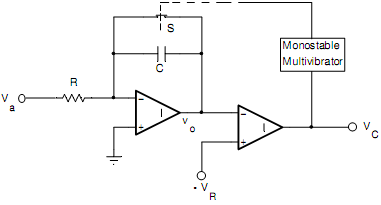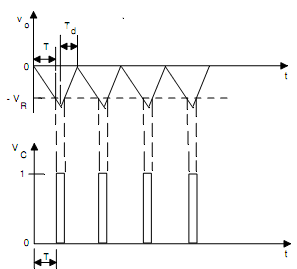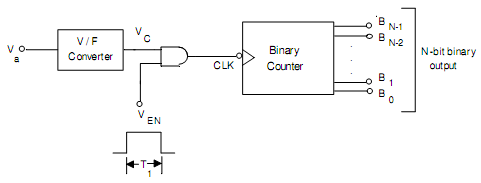## Draw the circuit of A/D converter using a V/F converter, Computer Engineering

Assignment Help:

Draw the schematic circuit of an Analog to Digital converter using Voltage-to Frequency conversion and explain its principle of operation. Draw its relevant Waveforms.

Ans:

Analog to Digital Converter Using Voltage-to-Frequency Conversion: An analog voltage can be converted into digital form by producing pulses whose frequency is proportional to the analog voltage. These pulses are counted by a counter for a fixed duration and the reading of the counter will be proportional to the frequency of the pulses and hence to the analog voltage.

A voltage-to-frequency converter is shown in Fig. (a). The analog voltage Va is applied to an integrator whose output is applied at the inverting input terminal of a comparator. The non-inverting input terminal of the comparator is connected to a reference voltage -VR.

Initially, the switch S is open and the voltage vo decreases linearly with time (vo = Vat/τ ) which is shown in Fig. 10(b). When the decreasing vo reaches -VR at t =T, the comparator output  VC   goes  HIGH.  This  is  used  to  close  the  switch  S  through  a  monostable multivibrator. When the switch S is closed, the capacitor C discharges, thereby returning the integrator output vo  to 0. Since the pulse width of the waveform VC is very small, therefore, a monostable multivibrator is used to keep the switch S closed for a sufficient time to discharge the capacitor completely. The rate at which the capacitor discharges depends upon the resistance of the switch.

Let the pulse width of the monostable multivibrator be Td. Therefore, the switch S remains closed for Td after which it opens and vo starts decreasing again.

If the integration time T >> Td, the frequency of the waveforms Vo and VC is given by

f = 1/( T + Td)  ≅  1/T = (1/ τ) (Va/VR)

Therefore we get an output waveform whose frequency is proportional to the analog input voltage. Using the voltage-to-frequency (V/F) converter an A/D converter is demonstrated in Fig.(c). The output of the voltage-to-frequency converter is applied at the clock (CK) input of a counter throughout an AND gate. For a fixed time interval T1, the AND gate is enabled. The reading of the counter at t = T1 is specified by

n =  fT1 =  (1/ τ) (Va/VR) T1  that is proportional to VaFig.(a) Logic diagram of Voltage-to-Frequency ConverterFig.(b) Waveforms of Voltage-to-Frequency ConverterFig.(c) Schematic circuit of A/D converter using a V/F converter

#### Write the benefits of broadcast model approach, a. Determine the non-functi...

a. Determine the non-functional system requirements that might be chosen for an application for a particular style and structures? b. Write the benefits of broadcast model appro

#### Prepurchase and purchase consummation of consumer mercantile, Differentiate...

Differentiate between Prepurchase and Purchase Consummation of consumer mercantile model. The prepurchase interaction for consumers comprises three activities: Product/ser

#### Performance of computer system, Performance of computer system: Comput...

Performance of computer system: Computer performance is frequently described in terms of clock speed (usually in MHz or GHz). It refers to the cycles per second of the main cl

#### Quiz help, which two class die class contain in computer programing.

which two class die class contain in computer programing.

#### When recursion functions are declared inline, Describe what happens when re...

Describe what happens when recursion functions are declared inline? Ans) Inline functions property says whenever it will call; it will copy the whole definition of that functi

aws hosting

#### What is optical mark recognition, Optical mark recognition (OMR)  OMR ...

Optical mark recognition (OMR)  OMR technology scans a printed form and reads pre-defined positions (where specific fields have been filled in for instance ?-?or _); system re

#### Which class used for multicasting in ip addressing scheme, In IP addressing...

In IP addressing scheme, class used for multicasting is: A class used for multicasting in IP addressing scheme is class D.

#### the entire pcd division, Will executing  SAP R/3 across the entire PCD div...

Will executing  SAP R/3 across the entire PCD division give the division with a competitive benefit?  Clarify  your answer carefully.

#### What are the advantagesof fact finding, What are the Advantagesof fact find...

What are the Advantagesof fact finding - Analyst obtains reliable data - It's possible to see exactly what is being done -  This is an inexpensive method in comparison o